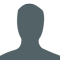## Twitter Sentiment Analysis using Python ProgramPavitra

Updated on 16-May-2022 12:23:58

In this article, we will be learning about the twitter sentimental analysis. We will register for twitter oAuth API, install all the dependencies and finally write our sentimental analyzer script.An API(Application programming interface) is a gateway that allows you to access some servers(Twitter) internal functionality.The prerequisite is that we have ... Read More

## Python program to interchange first and last elements in a listPavitra

Updated on 11-Jul-2020 12:01:53

In this article, we will learn about the solution to the problem statement given below.Problem statement − We are given a list, we need to swap the last element with the first element.There are 4 approaches to solve the problem as discussed below−Approach 1 − The brute-force approachExample Live Demodef swapLast(List):   ... Read More

## Python program to multiply two matricesPavitra

Updated on 11-Jul-2020 12:00:37

In this article, we will learn about the solution to the problem statement given below.Problem statement − We are given two matrices, we need to multiply them and print the result.For two matrices to be multiplied columns of the first matrix must be identical to that of the rows of the ... Read More

## Python program to find the sum of all items in a dictionaryPavitra

Updated on 11-Jul-2020 11:56:02

In this article, we will learn about the solution to the problem statement given below.Problem statement − We are given a dictionary, and we need to print the 3 highest value in a dictionary.Three approaches to the problem statement are given below:Approach 1 − Calculating sum from the dictionary iterableExample Live Demo# ... Read More

## Count words in a sentence in Python programPavitra

Updated on 11-Jul-2020 11:33:33

In this article, we will learn about the solution to the problem statement given below.Problem statement − We are given a string we need to count the number of words in the stringApproach 1 − Using split() functionSplit function breaks the string into a list iterable with space as a delimiter. ... Read More

## Python program to create a dictionary from a stringPavitra

Updated on 11-Jul-2020 11:32:35

In this article, we will learn about the solution to the problem statement given below.Problem statement − We are given a string input, we need to convert it into dictionary typeHere we will discuss two methods to solve the problem without using a built-in dict() function.Method 1 − Using eval() methodEval ... Read More

## Python program to find occurrence to each character in given stringPavitra

Updated on 11-Jul-2020 11:31:49

In this article, we will learn about the solution to the problem statement given below.Problem statement − We are given a string, we need to find the occurrence of each character in a given string.Here we will be discussing 3 approaches as discussed below:LApproach 1 − The brute-force approachExample Live Demotest_str = ... Read More

## Python program to find the second largest number in a listPavitra

Updated on 11-Jul-2020 11:30:42

In this article, we will learn about the solution to the problem statement given below.Problem statement − We are given a list, we need to display the second largest number in a list.There are three approaches to solve the problem−Approach 1 − We use the set() function & remove() functionExample Live Demolist1 ... Read More

## Python program to find the second maximum value in DictionaryPavitra

Updated on 11-Jul-2020 11:28:51

In this article, we will learn about the solution to the problem statement given below.Problem statement − We are given two integers, we need to print the second maximum value in the dictionaryNow let’s observe the concept in the implementation below−Approach 1 − Using sorted() function by negative indexesExample Live Demo#input example_dict ... Read More

## Python program to Count Even and Odd numbers in a ListPavitra

Updated on 11-Jul-2020 11:25:39

In this article, we will learn about the solution to the problem statement given below.Problem statement − We are given a list, we need to count even and odd numbers in a list.There are three methods as discussed below−Approach 1 − Using brute-force approachExample Live Demolist1 = [21, 3, 4, 6, 33, ... Read More

1 2 3 4 5 ... 24 Next